#Higher Order Linear Equations: Introduction and Basic ResultsLet us consider the equation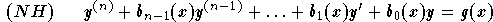,

and its associated homogeneous equation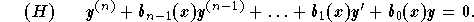The following basic results hold:

(1)
Superposition principle
Let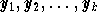be solutions of the equation (H). Then, the function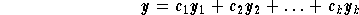is also solution of the equation (H). This solution is called a linear combination of the functions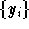;

(2)
The general solution of the equation (H) is given by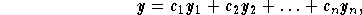where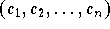are arbitrary constants and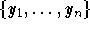are n solutions of the equation (H) such that,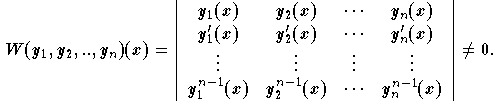In this case, we will say thatare linearly independent. The functionis called the Wronskian of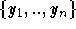. We have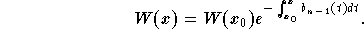Therefore,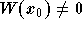, for some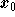, if and only if,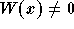for every x;

(3)
The general solution of the equation (NH) is given by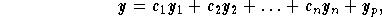whereare arbitrary constants,are linearly independents solutions of the associated homogeneous equation (H), and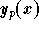is a particular solution of (NH).[Differential Equations] [First Order D.E.] [Second Order D.E.]
[Geometry] [Algebra] [Trigonometry ]
[Calculus] [Complex Variables] [Matrix Algebra]S.O.S MATHematics home page

Do you need more help? Please post your question on our S.O.S. Mathematics CyberBoard.Author: Mohamed Amine Khamsi